# Grid Plot in Python using Seaborn

• Last Updated : 22 Jun, 2020

Grids are general types of plots that allow you to map plot types to grid rows and columns, which helps you to create similar character-separated plots. In this article, we will be using two different data sets (Iris and Tips) for demonstrating grid plots

## Using Iris Dataset

We are going to use the Iris dataset which is a very famous dataset available as an in-built dataset. It contains a measurement of a bunch of different Irises(flowers). The dataset comprises of four measures: sepal distance, sepal weight, petal length, and petal width. We will be using the following code snippet for all the examples used below.

Code Snippet:

## Python3

 `import` `seaborn as sns ` `import` `matplotlib.pyplot as plt ` ` `  ` `  `iris ``=` `sns.load_dataset(``"iris"``) ` `iris.head() ` ` `  `# YOUR CODE HERE`

Output: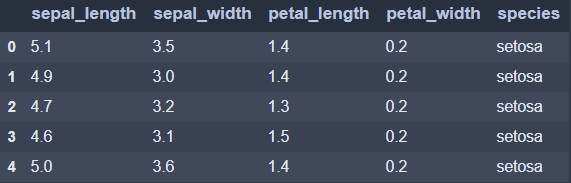Note: Place the below code snippets at the place of the YOUR CODE HERE.

#### Pair Plot

Pair Plot is like an automated joint plot for the entire dataset. In a sample, a pair plot maps a pairwise partnership. The `pairplot()` method generates an Axes map, such that each data vector is spread over a single row in the y-axis and across a single column in the x-axis. That generates plots as shown below.

```sns.pairplot(data = iris)
```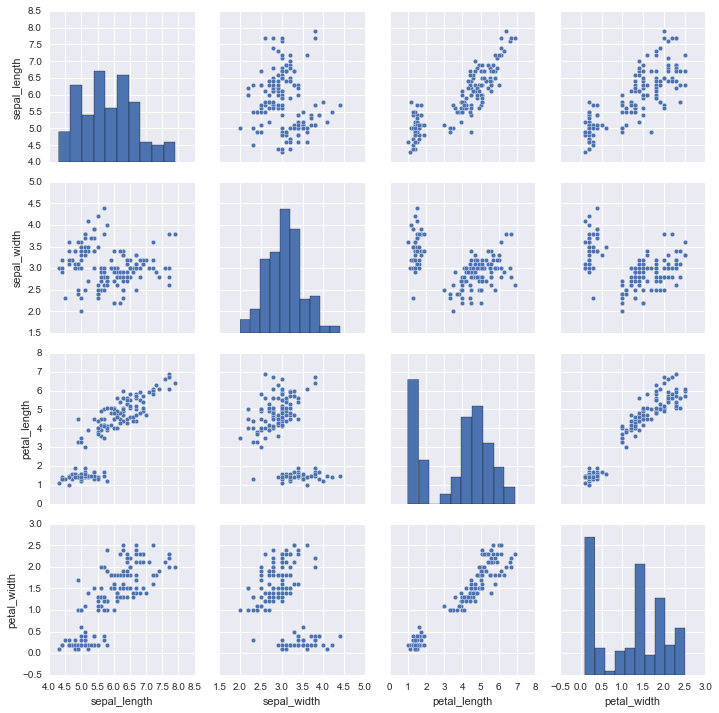We use ‘hue’ to visualize independent color for each type of ‘species’ in the plot. And the palette is used for customizing colors of the plot as shown below.

```sns.pairplot(iris, hue="species", palette="rainbow")
```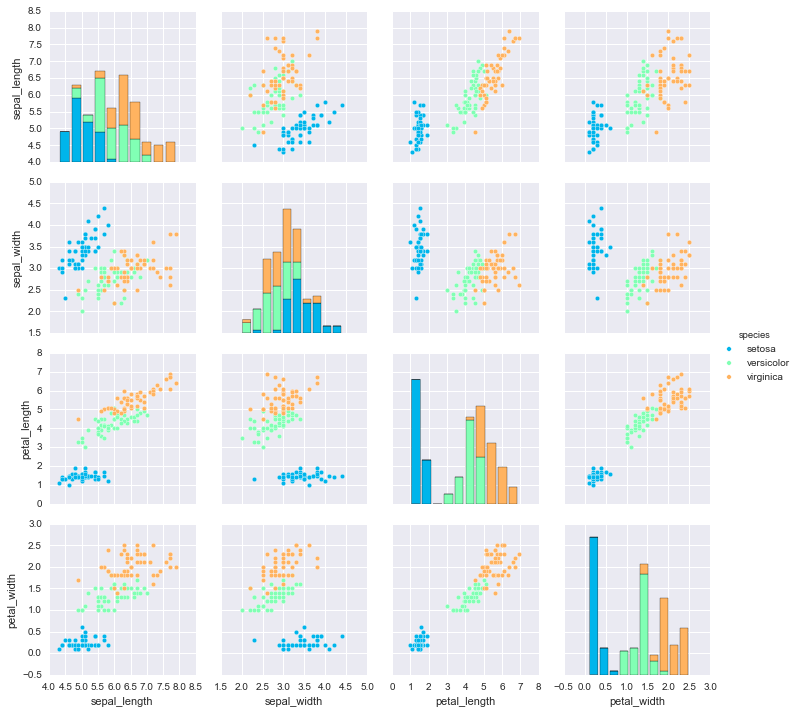#### Pair Grid

We can customize pair plot by using seaborn’s PairGrid mechanism. PairGrid takes all the numerical columns and grids them up making subplots as shown below.

```sns.PairGrid(data = iris)
```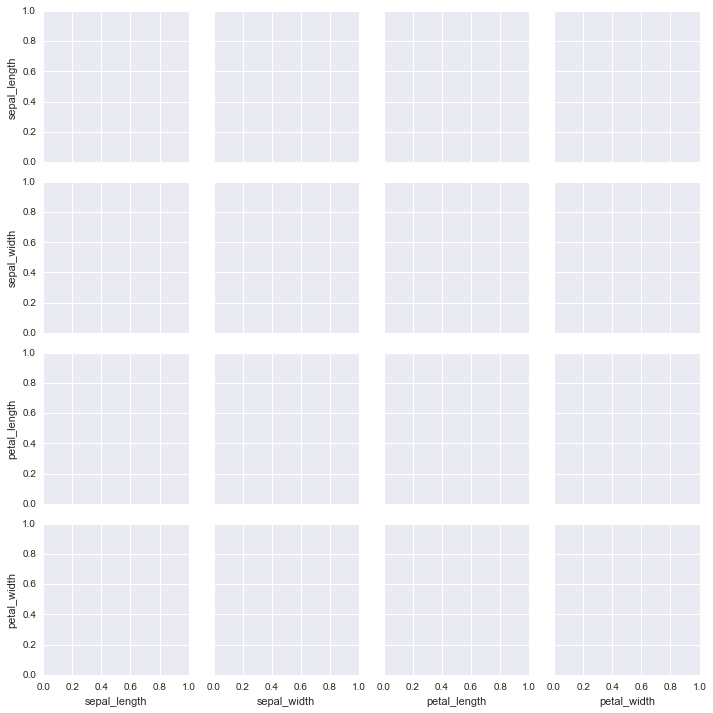We can map to the grid by calling ‘.map’ off of it. Over here we have called the scatter plot by ‘.scatter’. Now all the grids will be of scatter plot kind. We can define the type of plot we want in the grids using the ‘.map’.

```g = sns.PairGrid(iris)
g.map(plt.scatter) #All the grids plot scatter plot
```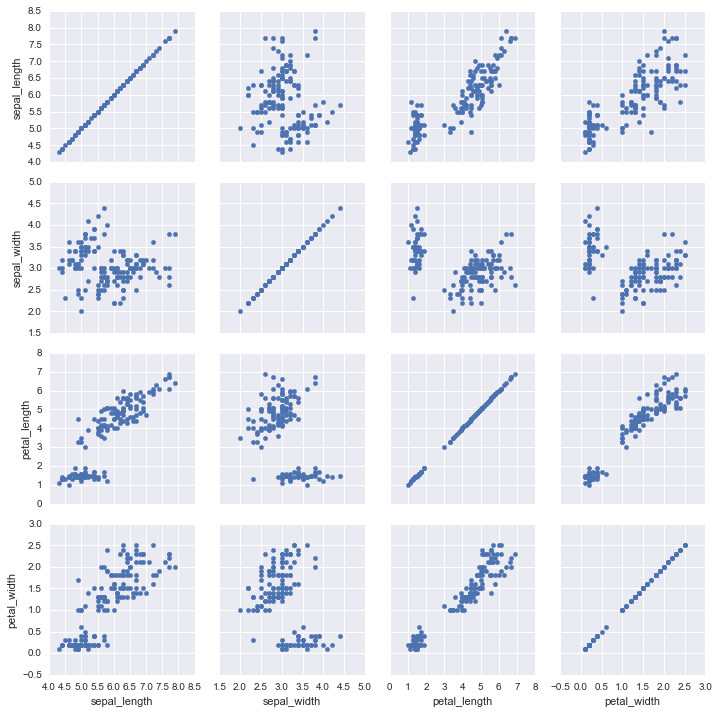To map into the upper grid, lower grid and diagonal grid we call ‘.map_upper’, ‘.map_lower’, ‘.map_diag’ off of the PairGrid. Over here we can see the diagonals are ‘hist’ type plot, the upper grid is’scatter’ type plot and the lower grid is ‘kde’ type plot.

```g = sns.PairGrid(iris)
g.map_diag(plt.hist)
g.map_upper(plt.scatter)
g.map_lower(sns.kdeplot)
```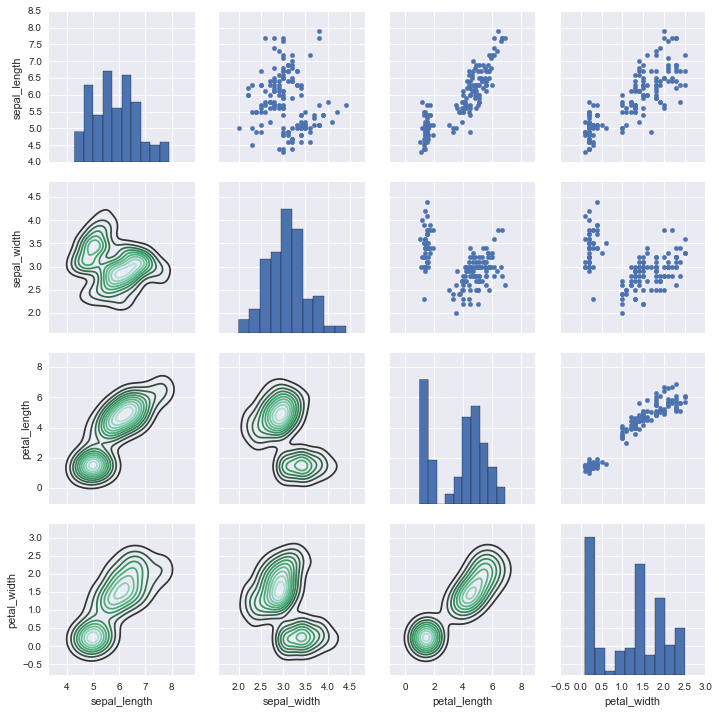## Using Tips Dataset

We are going to use another in-built dataset. The dataset comprises of seven features: total bill, tip, sex, smoker, day, time, size. We will be using the following code snippet for all the examples used below.

Code Snippet:

## Python3

 `import` `seaborn as sns ` `import` `matplotlib.pyplot as plt ` ` `  ` `  `tips ``=` `sns.load_dataset(``"tips"``) ` `tips.head() ` ` `  `# YOUR CODE HERE`

Output: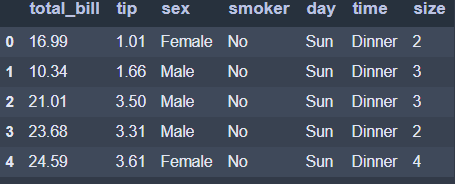Note: Place the below code snippets at the place of the YOUR CODE HERE.

#### FacetGrid

FacetGrid is a general way of creating plot grids based on a function. Its object uses the dataframe as input and the names of the variables that shape the row, column, or color dimensions of the grid.

Over here we can see, as there are two types of values in the smoker column which is smoker = No and smoker = Yes so this creates two rows in grid one for smoker = Yes and other for smoker = No. For the columns, as there are two types of values in time column which is time = Lunch and time = Dinner so this creates two columns in grid one for time = Lunch and other for time = Dinner.

```g = sns.FacetGrid(tips, col="time", row="smoker")
```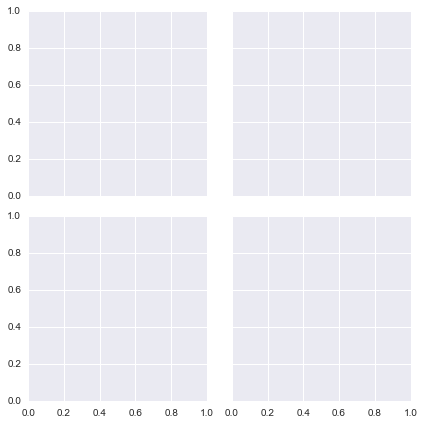The total bills are plotted as hist across the grid we created using’map’.

```g = sns.FacetGrid(tips, col="time",  row="smoker")
g = g.map(plt.hist, "total_bill")
```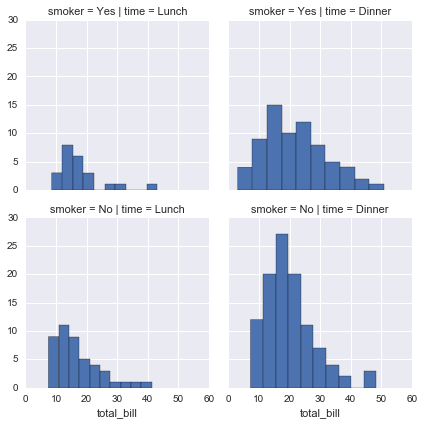Over here we have defined hue as sex and also plotted a scatter plot where X-axis is total_bill and Y-axis is yip..

```g = sns.FacetGrid(tips, col="time",  row="smoker", hue='sex')
g = g.map(plt.scatter, "total_bill", "tip").add_legend()
```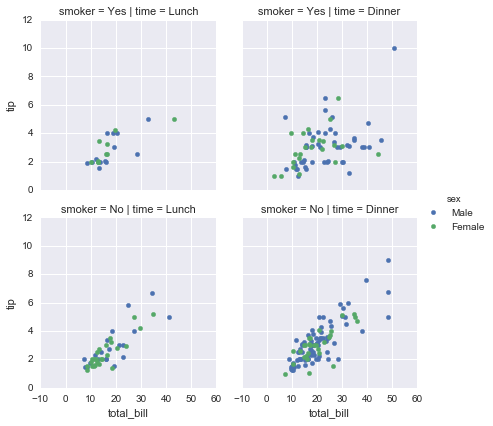Joint Grid

JointGrid is the general version for the grid type of `jointplot()`. Jointplot by Seaborn shows a relationship in the margins between 2 variables (bivariate) and 1D profiles (univariate). This plot is a product form that wraps up the JointGrid.

```g = sns.JointGrid(x="total_bill", y="tip", data=tips)
```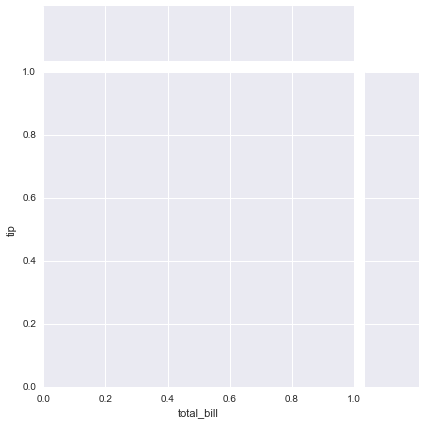```g = sns.JointGrid(x="total_bill", y="tip", data=tips)
g = g.plot(sns.regplot, sns.distplot)
```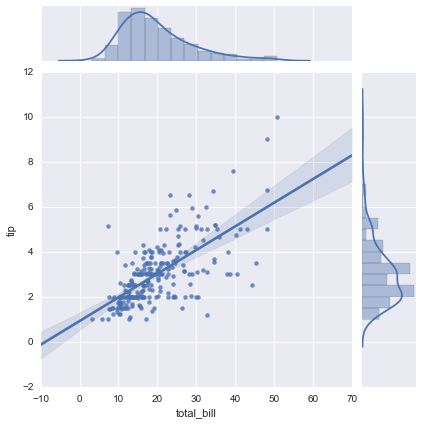My Personal Notes arrow_drop_up
Recommended Articles
Page :